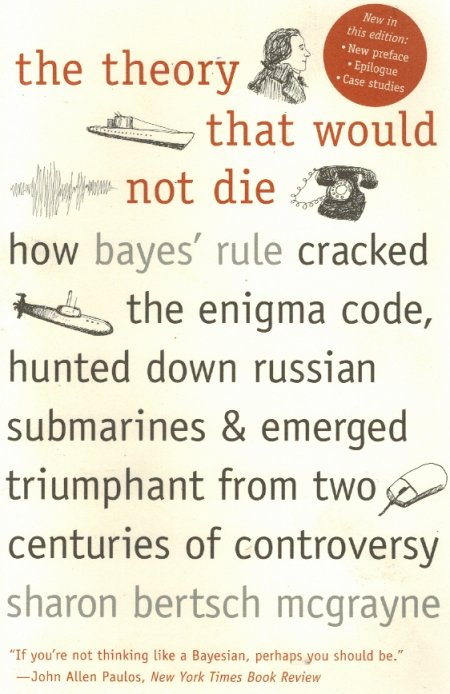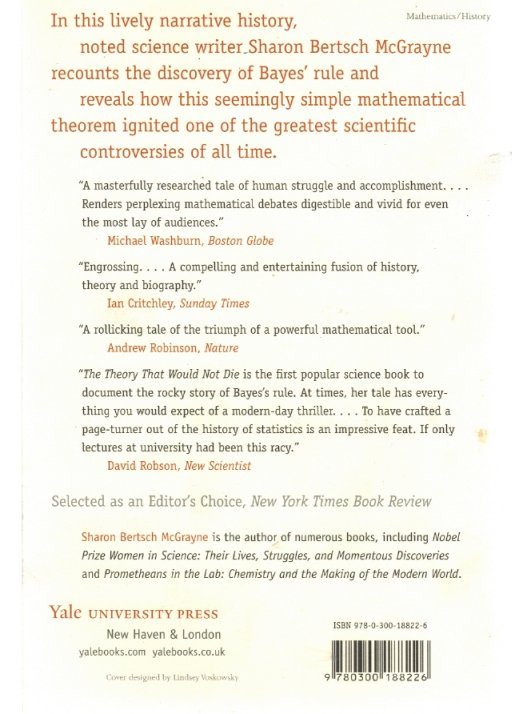# Why do people use Bayesian methods?

## Recommended Posts

I have done empirical research for 10+ years and I'd say I'm familiar with statistical methods, both Bayesian and classical. So, I have a question:

Why do people use Bayesian methods?

Can anybody give a good reason? I'm all ears because I can give three obvious disadvantages:

• They're damn slow.
• People don't really check that the Markov chains have converged. (Ideally, you should do this by formal statistics like effective sample size, but most people just eyeball the trace and the scientific journals don't require formal tests.)
• Nobody checks how the prior affects the results. Again, the journals don't require you to do this, and you just use the prior which gives the best convergence.

I think that Bayesian methods should be dumped out of the window and replaced by the good old maximum likelihood. Show me that I'm wrong!

##### Share on other sites

I learnt classical stats so i'm in no position to defend Bayesian approaches, but i'm not sure we need to dump Bayesian techniques. They are both tools and some jobs may be better suited to one or the other. I know there are a lot of physicists on this site so i wonder what they would make of a Bayesian approach to something like quantum theory.

Also, something i've never understood: both approaches are consistent with the Kolmogorov axioms, so in what sense are they really different at a fundamental level?

I'm surprised this topic hasn't reared its head on this forum before.

##### Share on other sites

6 hours ago, To_Mars_and_Beyond said:

I have done empirical research for 10+ years and I'd say I'm familiar with statistical methods, both Bayesian and classical. So, I have a question:

Why do people use Bayesian methods?

Can anybody give a good reason? I'm all ears because I can give three obvious disadvantages:

• They're damn slow.
• People don't really check that the Markov chains have converged. (Ideally, you should do this by formal statistics like effective sample size, but most people just eyeball the trace and the scientific journals don't require formal tests.)
• Nobody checks how the prior affects the results. Again, the journals don't require you to do this, and you just use the prior which gives the best convergence.

I think that Bayesian methods should be dumped out of the window and replaced by the good old maximum likelihood. Show me that I'm wrong!

What is the probability that tomorrow a meteorite will strike New York, killing every inhabitant ?

Classically this event has never happened so has a P value of zero.

32 minutes ago, Prometheus said:

I know there are a lot of physicists on this site so i wonder what they would make of a Bayesian approach to something like quantum theory.

The probability that both Bayes and Classical theory work with is the same quantity.

It is the estimation of this probability that differs, neither is 'better' than the other, each have their areas of maximum suitability.

##### Share on other sites

13 hours ago, studiot said:

What is the probability that tomorrow a meteorite will strike New York, killing every inhabitant ?

Classically this event has never happened so has a P value of zero.

The probability that both Bayes and Classical theory work with is the same quantity.

It is the estimation of this probability that differs, neither is 'better' than the other, each have their areas of maximum suitability.

This is true. In extremely small samples, you can't do classical aka. frequentist analysis. Another example is a coin toss. If you toss a coin only once and get heads, classical statistics says that the probability of heads is 1.00 and there's no uncertainty to it. But are this kind of examples really very realistic? Who would do serious statistical analysis from 1, 2 or 3 observations? And let us think of your New York example again... As you say, there's no data, so aren't you just analyzing your prior? I'd say this is more sort of probability, not statistics.

As both of you say, @Prometheus and @studiot, both Bayesian and classical statistics work on probability and are consistent with Kolmogorov axioms. The difference in nutshell:

• Bayesian statistics: Parameter has a prior distribution p(a). After observing data, it has a posterior distribution p(a|y).
• Classical statistics: The parameter has a true value a*. We can calculate from data an estimator a^ which tends to the true value, subject to certain assumptions. In practice, the estimator a^ will have some distribution p(a^). We work with this distribution in just the same way as we would with the posterior p(a|y), i.e. we calculate confidence intervals.

My point is that calculating the classical confidence intervals is much faster and more reliable than doing the MCMC to analyze the posterior.

##### Share on other sites

6 minutes ago, To_Mars_and_Beyond said:

This is true. In extremely small samples, you can't do classical aka. frequentist analysis. Another example is a coin toss. If you toss a coin only once and get heads, classical statistics says that the probability of heads is 1.00 and there's no uncertainty to it. But are this kind of examples really very realistic? Who would do serious statistical analysis from 1, 2 or 3 observations? And let us think of your New York example again... As you say, there's no data, so aren't you just analyzing your prior? I'd say this is more sort of probability, not statistics.

As both of you say, @Prometheus and @studiot, both Bayesian and classical statistics work on probability and are consistent with Kolmogorov axioms. The difference in nutshell:

• Bayesian statistics: Parameter has a prior distribution p(a). After observing data, it has a posterior distribution p(a|y).
• Classical statistics: The parameter has a true value a*. We can calculate from data an estimator a^ which tends to the true value, subject to certain assumptions. In practice, the estimator a^ will have some distribution p(a^). We work with this distribution in just the same way as we would with the posterior p(a|y), i.e. we calculate confidence intervals.

My point is that calculating the classical confidence intervals is much faster and more reliable than doing the MCMC to analyze the posterior.

"But are this kind of examples really very realistic?"

Yes a famous example is the hunt for the Thresher.

"classical statistics says that the probability of heads is 1.00 and there's no uncertainty to it"

That's one way to put it.

Of course there are at least three different interpretations of a probability of 1 or 0.

Another interesting observation is that Bayes theory predated 'classical' theory.

There is an interesting book about the history and many modern applications of Bayes theory.
I will have to dig out the details for you.

##### Share on other sites

Save your efforts, @studiot, I have seen too many Bayes textbooks.

I'd be happy to hear your three interpretations of probability. In statistics, you have typically the two explanations: A frequentist interpretation means that if you repeat the same experiment infinitely many times you will get a proportion P (so think of your coin toss, you'd always get heads). A subjectivist interpretation means that probability is our measure of ignorance.

In Kolmogorov axioms, probability is just a measure defined on some abstract space and it induces measures on all variables of interest, be they vectors or scalars. So probability is just some number, and there's no point in asking what it really is or how it relates to the real world.

Edited by To_Mars_and_Beyond
##### Share on other sites

23 minutes ago, To_Mars_and_Beyond said:

Save your efforts, @studiot, I have seen too many Bayes textbooks.

But have you seen this one ?So what does a probability, P(E)=1 ,  of 1 mean ?

1) Well with a strictly a priori approach it means that E must always occur

2 Using an empirical (objective approach) it means that E has always occurred, but does not imply that E will occur in the future.

3) Using a subjective approach it means that we think E will occur, but does not mean it must occur.

The last one is used by bookies to set their odds at races etc.

##### Share on other sites

In my books P(E)=1 means infinite odds.

1) I'm ok with that.

2) To me is that we have a frequentist estiamate P(E)^=1 and we admit that we don't believe in our own estimate.

3) To me is just lazy thinking.

##### Share on other sites

12 minutes ago, To_Mars_and_Beyond said:

In my books P(E)=1 means infinite odds.

1) I'm ok with that.

2) To me is that we have a frequentist estiamate P(E)^=1 and we admit that we don't believe in our own estimate.

3) To me is just lazy thinking.

Then I would say you don't fully understand probability.

##### Share on other sites

• 3 weeks later...
On 3/13/2021 at 11:55 PM, studiot said:

What is the probability that tomorrow a meteorite will strike New York, killing every inhabitant ?

hahahahahOn 3/15/2021 at 1:02 PM, To_Mars_and_Beyond said:

P(E)=1 means

that is just a theoretic part, and simply shows the summation of all possible probabilities. So ,equal to 1.

Edited by ahmet
##### Share on other sites

1 hour ago, ahmet said:

and simply shows the summation of all possible probabilities.

I'm sure you don't quite mean that.

🙂

##### Share on other sites

51 minutes ago, studiot said:

I'm sure you don't quite mean that.

🙂

yes , to say: "the probability for whole domain set of random variable " might be better.

in fact, I do not believe the ncertainity when we locate us in mathematics. because everything is definite/certain.

the uncertain parts are most probably just belong to our failures.

haha ,what I have written , like phylosophistsEdited by ahmet

## Create an account

Register a new account